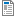National Curriculum

# Reception/Year 1 Maths Plans

Hamilton provide mixed Reception/Year 1 weekly maths plans (below). We hope, in time, to develop maths blocks for this mixed year combination. Find out more about our plans to phase out these mixed age plans in favour of Year R/1 maths blocks which we believe offer all the advantages of the weekly plans and more.

Supporting documents for set
• Week
• Title
1
+ Details
Number and place value

Reception: Objectives: Recite numbers to 20 then 100; Recognise, read and begin to write numbers more and less than 10; Count up and back from 20 to zero; Order numbers to 20 by placing them on a line; Place three numbers up to 20 in order. Year 1: Objectives: Find one more and one less than 2-digit number; Count in 10s from 10; Count in tens from any number; Estimate a quantity; Find ten more and ten less than any 2-digit number.Spring Week 1 Plan
2
+ Details

Reception Objectives: Know ordinal numbers; Count actions and sounds - recite numbers to 20 and then to 100; Place numbers 1-20 on a number line; Place three numbers up to 20 in order; Say the next number to a given number up to 10; match numerals to spoken numbers. Year 1: Objectives: Know number bonds to 8; Know number bonds to 9; Recognise that adding can be done in any order; Find doubles to double 6; Add three numbers using number bonds to 10 and doubles. Includes bar model examples.Spring Week 2 Plan
3
+ Details
Money and number

Reception objectives: Recognise 1p, 2p, 5p and 10p coins; Use money in shopping using 1p, 2p, 5p and 10p coins; Solve practical problems involving counting or role-play. Year 1 objectives: Recognise 1p, 2p, 5p, 10p, £1 coins; Find totals using more than two coins up to 10p in value; Work out what coins can be used to pay an amount up to 10p; Begin to find all possibilities by making an ordered list; Count on in tens from single-digit numbers and back; Relate counting on in tens to find 10 more/less than any two-digit number.

4
+ Details
Measures and shape

Reception Objectives: Compare two weights using direct comparison, use language of heavier and lighter than; Use uniform non-standard units to measure weights up to 10 units; Know how key times of day (hours only) are shown on analogue and digital clocks. Year 1: Objectives: Estimate, measure and compare objects, choosing and using suitable uniform non-standard or standard units; Use vocabulary related to time; Read the time to the hour (o’clock) and half past the hour.

5
+ Details
Number and beginning of multiplication

Reception objectives: Count to 20 then to 100; match numbers 1-9 to sets and recognise zero as the empty set; Count back from 20 to 0; Compare numbers to 20; Read numbers to 20; match numbers to sets. Year 1 objectives: Count in tens; Count in twos; Recognise number sequences; Know odd and even numbers; Double numbers to 10, halve even numbers to 20; Use sorting diagrams.

6
+ Details

Reception objectives: Say the next number, without counting from 1; Add 1 to any number up to 10, say the corresponding addition; Add 2 to any number up to 10, say the corresponding addition. Year 1 objectives: Know what each digit means in a 2-digit number, partition 2-digit numbers into tens and units; Order numbers to 100 on a track, then a beaded line; Compare two numbers less than 100, say which is more or less; Say a number between any given neighbouring pairs of multiples of ten (e.g. 40 and 50); Investigate and create different 2-digit numbers.

7
+ Details

Reception objectives: Find one more and two more than any number to 10. Begin to find 1 more than a number up to 20. Begin to find small differences (using ‘it’s not fair’). Year 1 objectives: Measure objects using non-standard units of measurements (cubes); Estimate and compare lengths: Find a difference in height/length; Find numbers with a given difference; Begin to use a systematic way of recording results.

8
+ Details
Shape and measures

Reception objectives: Begin to name and describe 3-D shapes. Begin to name and describe a cube, cuboid and sphere. Use 3-D shapes to make models. Use 3-D shapes to print and describe the 2-D shapes printed. Revise 2-D shapes and their properties. Year 1 objectives: Name common 3D shapes and their faces. Name, describe and sort common 3D shapes. Describe common 3D shapes. Read the time to the half hour on analogue clocks and digital clocks.

9
+ Details

Reception objectives: Find different ways to partition sets of 5 objects; Read the corresponding addition. Find different ways to partition sets of 6 objects; Read the corresponding addition. Early subtraction, guess how many are hiding. Early subtraction, guess how many are left on the bus (count back) Year 1 objectives: Find pairs to 6, 7, 8, 9 and 10; Begin to relate addition and subtraction facts; Find doubles and near doubles; Add 10 then small multiples of 10 to 2-digit numbers; Subtract 10 and then small multiples of 10 from 2-digit numbers. Includes bar model examples.

10
+ Details
Addition, subtraction and measures, shape and data

Reception objectives: Find different ways to partition sets of 10 objects and read corresponding additions. Begin to recognise coins and sort coins into sets. Sort 3D shapes according to whether they roll or not, stack or not. Year 1 objectives: Know number bonds to 10 and use pairs to ten to bridge ten (8+2, 8+3…) with visual support; Add single-digit amounts of pence, bridging 10p; Sort calculations according to whether they will bridge ten or not. Includes bar model examples.

11
+ Details